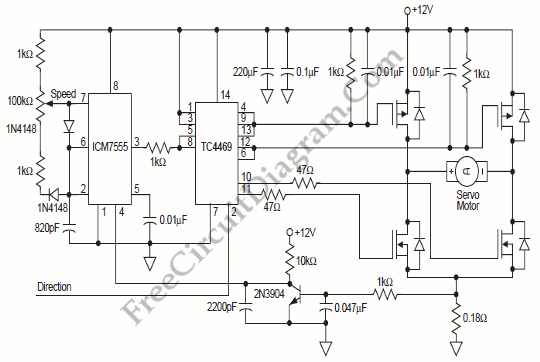## Interfacing Triacs with Logic Inputs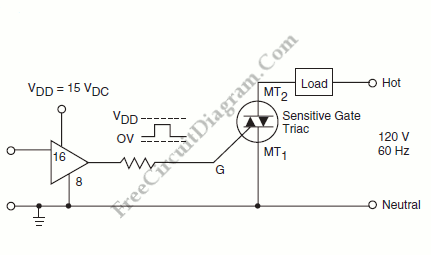One easy way if load current is approximately 5 A or less is shown on figure below. The sensitive gate triac acts as a direct power switch controlled by TTL, HTL, CMOS, or integrated circuit operational amplifier. The triac can be activated by a timed pulse from the system’s logic from anywhere in the AC sinewave producing a phase-controlled load. […]

## Temperature Measurement (Sensing) and Monitoring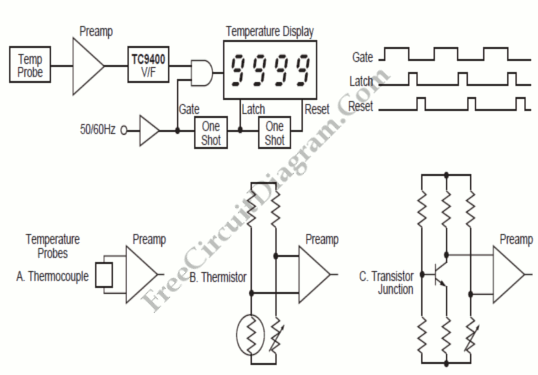Temperature  measurement (sensing) and monitoring will be economical and straightforward if we apply the input of  TC9400 V/F converter with a voltage from output of a probe, such as shown in diagram below. The V/F output is simply counted to display the temperature. The TC9400 can be used to modulate an RF transmitter for a long distance data transmission. The […]

## Proportional FLow Rate Controller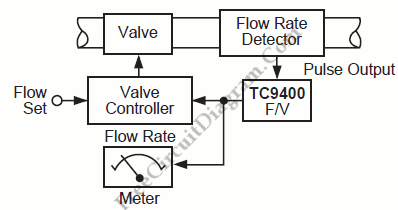Process industries deal with various control systems, and fluid mass transfer control is one of them. We can use a TC9400 F/V converter to regulate the amount of liquid or gas flowing through a pipeline. A pulse train whose frequency is proportional to the rate of flow through it is generated by the flow rate detector. This frequency is converted […]

## Simple Proportional-Integral Motor Speed Control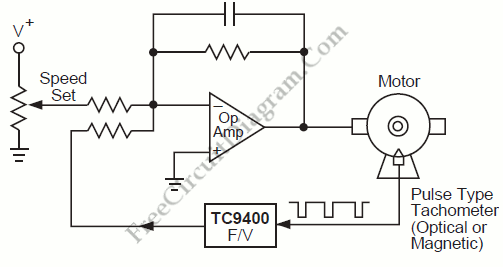Another example of frequency-to-voltage  converter application similar with RPM/Speed Indication is motor speed control. Here the converter is used to measure the actual speed which will be fed back to keep the actual speed stabilized according to a reference. Here is the block diagram of this control system: If the speed is to high, the output of the F/V converter […]

## RPM/Speed Indication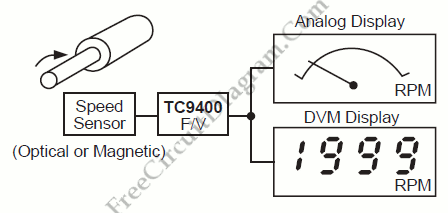One example of frequency-to-voltage converter application is RPM/Speed indication, converting pulse signal from speed sensor into a linear voltage to drive analog meter. Here is the  block diagram of such speed indication system: Since they measure the number of events per time period, flow rates and revolutions per second are nothing more than frequency signals. This flow and revolution will […]

## Stereo VU Booster Circuit using Transistors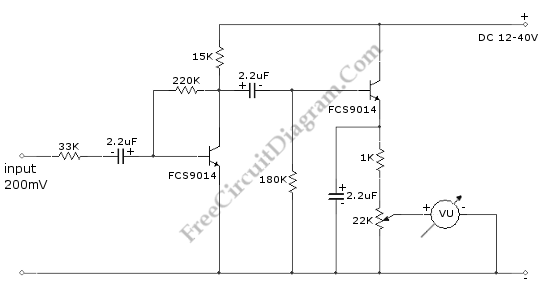This is a Stereo VU Booster circuit. In this circuit,  shown in the schematic diagram, transistor FCS9014 is used. Actually you can use almost any type of small signal NPN transistor. This transistor usually used for regulator and pre-amp circuit.  Here is the schematic diagram of the circuit: The schematic diagram show only one channel, so you have to build  […]